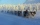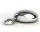Ophelia

Ophelia recorded the temperature of a cold store every two hours.
1. At 6am it was -4°C and at 8am it was -1°C. By how much did the temperature rise?
2. The temperature went up by 5°C in the next two hours. .What was the temperature at 10am?

Result

t1 =  3 °C
t2 =  4 °C

Solution:Leave us a comment of this math problem and its solution (i.e. if it is still somewhat unclear...):Be the first to comment!Next similar math problems:

1. LaboratoryMang Elio went to his laboratory at 7:00am And recorded that the temperature was 11.07°C. At lunchtime, the temperature rose by 27.9°C but dropped by 8.05°C at 5:00 PM. What was the temperature at 5:00 PM?
2. Temperature differenceThe highest temperature of Libya was recorded 58 degree Celsius and the lowest was recorded -88 degree Celsius, what is the temperature difference?
3. The temperartureThe temperarture is 0 degrees. It drops 5 degrees after 1 hour and then drops 3 degrees more the next hour. What is the temperature after 6 hours?
4. The temperature 3The temperature is -12 degrees Fahrenheit. During the following 5 hours the temperature decrease 23 degrees Fahrenheit what is the temperature at 11 pm?
5. Melting pointThe melting point of mercury is -36°F and its boiling point is 672°F. What is the difference between the boiling point and the melting point?
6. On SundayOn Sunday the temperature reached a high of 38° above 0. That night it dropped to 23° below 0. What is the difference between the high and low temperatures for Sunday?
7. 8 degrees8 degrees Celsius it cools down by 9 degrees Celsius. What is the resultant temperature?
8. NoontimeIn the morning temperature was 110°F. By noontime it has gone up by 15°F. What was the noon temperature. (Give your answer in °F)
9. The lowestThe lowest temperature on Thursday was 20°C. The lowest temperature on Saturday was 12°C. What was the difference between the lowest temperatures?
10. The temperature 2The temperature of Siberia fell13 degrees below 2/3 degrees centigrad. What is the temperature there now?
11. Temperature changeThe mean temperature change is -3.2°F per day for five days. What is the total change over the five day period?
12. Difference in temperaturesThe temperature in the number line show minus 15 degree celsius and 8 degree celsius. What is the different between the temperatures?
13. Mixing Celsius and FahrenheitAdd up three temperatures: 5°F +6°F +0°C
14. Bitoo and ReenaBitoo ate 3/5 part of an apple and the remaining part was eaten by his sister Reena. How much part of an apple did Renna eat? Who had the larger share? By how much?
15. Combine / add termCombine like terms 4c+c-7c
16. CupcakesIn a bowl was some cupcakes. Janka ate one third and Danka ate one quarter of cupcakes. a) How many of cookies ate together? b) How many cookies remain in a bowl? Write the results as a decimal number and in notepad also as a fraction.
17. Adding mixed numerals3 3/4 + 2 3/5 + 5 1/2 Show your solution.